# Journey 5

A man has to do a journey of 84km in 3 hours. He travels the first 30km at 20km/hr. At what rate must he travel the remaining distance to complete his journey on time?

Result

v =  36 km/h

#### Solution:

84 - 30 = v * (3 - 30/20)

30v = 1080

v = 36

Calculated by our simple equation calculator.Our examples were largely sent or created by pupils and students themselves. Therefore, we would be pleased if you could send us any errors you found, spelling mistakes, or rephasing the example. Thank you!

Leave us a comment of this math problem and its solution (i.e. if it is still somewhat unclear...):Be the first to comment!Tips to related online calculators
Check out our ratio calculator.
Do you have a linear equation or system of equations and looking for its solution? Or do you have quadratic equation?
Do you want to convert velocity (speed) units?

## Next similar math problems:

1. Two bodiesThe rectangle with dimensions 8 cm and 4 cm is rotated 360º first around the longer side to form the first body. Then, we similarly rotate the rectangle around the shorter side b to form a second body. Determine the ratio of surfaces of the first and seco
2. MilkThere were 22 liters of milk in three containers. There was 6 liters more in the first container than in the second. After pouring 5 liters from the first container into the third container, the same quantity of milk is in the second and third container.
3. Scholarship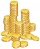The annual scholarship of the best student and second-best student in the class is 3500 euros in total. The best student scholarship in 8 months is the same as the second-best student scholar in the class for the whole year. How big is the annual scholars
4. A cloth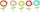There are flower cloth 495 meters, white cloth 330 meters. What is the percentage of white cloth? How much is white cloth less than flower cloth?
5. Unknown number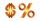15% of the unknown number is 18 less than 21% of the same number. What is the unknown number?
6. The product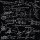The product of a number plus that number and its inverse is two and one-half. What is the inverse of this number
7. Cylinder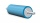The 1.8m cylinder contains 2000 liters of water. What area (in dm2) of this container is the water?
8. Pairs of socks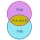Ferdinand has twelve pairs of socks, one sock is leaky. What is the probability of putting on a leaky sock?
9. Diagonal intersectisosceles trapezoid ABCD with length bases | AB | = 6 cm, CD | = 4 cm is divided into 4 triangles by the diagonals intersecting at point S. How much of the area of the trapezoid are ABS and CDS triangles?
10. Average monthly salaryA total of 10 teachers work at one small school in Moravia. The monthly salary of each is 21,500 CZK or 21,800 CZK or 22,500 CZK according to their education and age. The average monthly salary for this school's teacher is 21 850 CZK. How many teachers of
11. Axial section of the coneThe axial section of the cone is an isosceles triangle in which the ratio of cone diameter to cone side is 2: 3. Calculate its volume if you know its area is 314 cm square.
12. Cone sideCalculate the volume and area of the cone whose height is 10 cm and the axial section of the cone has an angle of 30 degrees between height and the cone side.
13. The Indian tent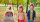The Indian tent is cone-shaped. Its height is 3.5 m. The diameter of the base is 2.5 m. How much canvas is needed to make a tire?
14. Baking muffins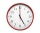Aunt Polly is baking muffins at a speed of 4 muffins per minute. Tom comes into the kitchen when Aunt Polly has 12 muffins, and begins to eat these muffins at a speed of 6 muffins per minute. When he finally leaves the kitchen, there are 4 muffins at the
15. Regular hexagonal pyramid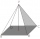Calculate the height of a regular hexagonal pyramid with a base edge of 5 cm and a wall height of w = 20cm. Sketch a picture.
16. Octagonal pyramidFind the volume of a regular octagonal pyramid with height v = 100 and the angle of the side edge with the plane of the base is α = 60°.
17. Tetrahedral pyramidDetermine the surface of a regular tetrahedral pyramid when its volume is V = 120 and the angle of the sidewall with the base plane is α = 42° 30´.